# Mean State

Period Mean (original grids) [K]
Model Period Mean (intersection) [K]
Model Period Mean (complement) [K]
Benchmark Period Mean (intersection) [K]
Benchmark Period Mean (complement) [K]
Bias [K]
RMSE [K]
Phase Shift [months]
Bias Score 
RMSE Score 
Seasonal Cycle Score 
Spatial Distribution Score 
Interannual Variability Score 
Overall Score 
Benchmark [-] 299.
CLM5PHSOFF [-] 299. 299. 300. 298. 300. 0.225 0.724 0.428 0.65 0.75 0.96 1.0 0.95 0.85
CLM5PHSON [-] 299. 299. 300. 298. 300. 0.285 0.690 0.366 0.67 0.78 0.97 1.0 0.96 0.86
Period Mean (original grids) [K]
Model Period Mean (intersection) [K]
Model Period Mean (complement) [K]
Benchmark Period Mean (intersection) [K]
Benchmark Period Mean (complement) [K]
Bias [K]
RMSE [K]
Phase Shift [months]
Bias Score 
RMSE Score 
Seasonal Cycle Score 
Spatial Distribution Score 
Interannual Variability Score 
Overall Score 
Benchmark [-] 300.
CLM5PHSOFF [-] 301. 301. 300. 300. 300. 0.460 0.798 0.168 0.75 0.86 0.99 0.99 0.96 0.90
CLM5PHSON [-] 301. 301. 301. 300. 300. 0.473 0.802 0.168 0.75 0.86 0.99 0.99 0.96 0.90
Period Mean (original grids) [K]
Model Period Mean (intersection) [K]
Model Period Mean (complement) [K]
Benchmark Period Mean (intersection) [K]
Benchmark Period Mean (complement) [K]
Bias [K]
RMSE [K]
Phase Shift [months]
Bias Score 
RMSE Score 
Seasonal Cycle Score 
Spatial Distribution Score 
Interannual Variability Score 
Overall Score 
Benchmark [-] 295.
CLM5PHSOFF [-] 296. 296. 297. 295. 297. 0.525 0.859 0.287 0.70 0.87 0.97 1.0 0.96 0.90
CLM5PHSON [-] 296. 296. 296. 295. 297. 0.505 0.854 0.273 0.71 0.87 0.97 1.0 0.96 0.89
Period Mean (original grids) [K]
Model Period Mean (intersection) [K]
Model Period Mean (complement) [K]
Benchmark Period Mean (intersection) [K]
Benchmark Period Mean (complement) [K]
Bias [K]
RMSE [K]
Phase Shift [months]
Bias Score 
RMSE Score 
Seasonal Cycle Score 
Spatial Distribution Score 
Interannual Variability Score 
Overall Score 
Benchmark [-] 262.
CLM5PHSOFF [-] 266. 262. 269. 262. 266. 0.253 1.14 0.0145 0.94 0.96 1.0 1.0 0.94 0.96
CLM5PHSON [-] 262. 261. 267. 262. 266. 0.258 1.13 0.0161 0.94 0.96 1.0 1.0 0.94 0.97
Period Mean (original grids) [K]
Model Period Mean (intersection) [K]
Model Period Mean (complement) [K]
Benchmark Period Mean (intersection) [K]
Benchmark Period Mean (complement) [K]
Bias [K]
RMSE [K]
Phase Shift [months]
Bias Score 
RMSE Score 
Seasonal Cycle Score 
Spatial Distribution Score 
Interannual Variability Score 
Overall Score 
Benchmark [-] 299.
CLM5PHSOFF [-] 299. 299. 300. 299. 299. 0.160 0.746 0.476 0.43 0.71 0.93 0.96 0.91 0.78
CLM5PHSON [-] 299. 299. 300. 299. 299. 0.226 0.750 0.486 0.42 0.72 0.93 0.96 0.91 0.78
Period Mean (original grids) [K]
Model Period Mean (intersection) [K]
Model Period Mean (complement) [K]
Benchmark Period Mean (intersection) [K]
Benchmark Period Mean (complement) [K]
Bias [K]
RMSE [K]
Phase Shift [months]
Bias Score 
RMSE Score 
Seasonal Cycle Score 
Spatial Distribution Score 
Interannual Variability Score 
Overall Score 
Benchmark [-] 271.
CLM5PHSOFF [-] 271. 271. 275. 271. 274. -0.0455 0.709 0.00842 0.97 0.97 1.0 1.0 0.98 0.98
CLM5PHSON [-] 271. 271. 275. 271. 274. -0.0249 0.647 0.00842 0.97 0.97 1.0 1.0 0.98 0.98
Period Mean (original grids) [K]
Model Period Mean (intersection) [K]
Model Period Mean (complement) [K]
Benchmark Period Mean (intersection) [K]
Benchmark Period Mean (complement) [K]
Bias [K]
RMSE [K]
Phase Shift [months]
Bias Score 
RMSE Score 
Seasonal Cycle Score 
Spatial Distribution Score 
Interannual Variability Score 
Overall Score 
Benchmark [-] 280.
CLM5PHSOFF [-] 281. 281. 289. 280. 285. 0.283 1.10 0.0208 0.90 0.96 1.0 1.0 0.97 0.96
CLM5PHSON [-] 281. 280. 288. 280. 285. 0.249 1.09 0.0222 0.90 0.96 1.0 1.0 0.96 0.96
Period Mean (original grids) [K]
Model Period Mean (intersection) [K]
Model Period Mean (complement) [K]
Benchmark Period Mean (intersection) [K]
Benchmark Period Mean (complement) [K]
Bias [K]
RMSE [K]
Phase Shift [months]
Bias Score 
RMSE Score 
Seasonal Cycle Score 
Spatial Distribution Score 
Interannual Variability Score 
Overall Score 
Benchmark [-] 297.
CLM5PHSOFF [-] 297. 297. 300. 297. 300. 0.162 1.07 0.164 0.76 0.88 0.98 1.0 0.95 0.91
CLM5PHSON [-] 297. 297. 300. 297. 300. 0.110 1.08 0.181 0.77 0.88 0.98 1.0 0.95 0.91
Period Mean (original grids) [K]
Model Period Mean (intersection) [K]
Model Period Mean (complement) [K]
Benchmark Period Mean (intersection) [K]
Benchmark Period Mean (complement) [K]
Bias [K]
RMSE [K]
Phase Shift [months]
Bias Score 
RMSE Score 
Seasonal Cycle Score 
Spatial Distribution Score 
Interannual Variability Score 
Overall Score 
Benchmark [-] 264.
CLM5PHSOFF [-] 264. 264. 268. 264. 264. 0.0749 0.718 0.0130 0.97 0.97 1.0 1.0 0.96 0.98
CLM5PHSON [-] 264. 264. 267. 264. 264. 0.0517 0.717 0.0135 0.97 0.97 1.0 1.0 0.96 0.98
Period Mean (original grids) [K]
Model Period Mean (intersection) [K]
Model Period Mean (complement) [K]
Benchmark Period Mean (intersection) [K]
Benchmark Period Mean (complement) [K]
Bias [K]
RMSE [K]
Phase Shift [months]
Bias Score 
RMSE Score 
Seasonal Cycle Score 
Spatial Distribution Score 
Interannual Variability Score 
Overall Score 
Benchmark [-] 295.
CLM5PHSOFF [-] 295. 295. 293. 295. 294. 0.473 0.695 0.0952 0.88 0.93 0.99 1.0 0.97 0.95
CLM5PHSON [-] 295. 295. 294. 295. 294. 0.479 0.699 0.0807 0.88 0.93 0.99 1.0 0.97 0.95
Period Mean (original grids) [K]
Model Period Mean (intersection) [K]
Model Period Mean (complement) [K]
Benchmark Period Mean (intersection) [K]
Benchmark Period Mean (complement) [K]
Bias [K]
RMSE [K]
Phase Shift [months]
Bias Score 
RMSE Score 
Seasonal Cycle Score 
Spatial Distribution Score 
Interannual Variability Score 
Overall Score 
Benchmark [-] 282.
CLM5PHSOFF [-] 282. 282. 285. 282. 286. 0.175 0.883 0.126 0.90 0.93 0.99 1.0 0.97 0.95
CLM5PHSON [-] 282. 282. 284. 282. 286. 0.117 0.866 0.145 0.90 0.94 0.99 1.0 0.97 0.96
Period Mean (original grids) [K]
Model Period Mean (intersection) [K]
Model Period Mean (complement) [K]
Benchmark Period Mean (intersection) [K]
Benchmark Period Mean (complement) [K]
Bias [K]
RMSE [K]
Phase Shift [months]
Bias Score 
RMSE Score 
Seasonal Cycle Score 
Spatial Distribution Score 
Interannual Variability Score 
Overall Score 
Benchmark [-] 287.
CLM5PHSOFF [-] 286. 287. 262. 287. 292. 0.236 0.910 0.143 0.83 0.90 0.99 1.0 0.96 0.93
CLM5PHSON [-] 283. 287. 249. 287. 292. 0.227 0.898 0.137 0.83 0.91 0.99 1.0 0.96 0.93
Period Mean (original grids) [K]
Model Period Mean (intersection) [K]
Model Period Mean (complement) [K]
Benchmark Period Mean (intersection) [K]
Benchmark Period Mean (complement) [K]
Bias [K]
RMSE [K]
Phase Shift [months]
Bias Score 
RMSE Score 
Seasonal Cycle Score 
Spatial Distribution Score 
Interannual Variability Score 
Overall Score 
Benchmark [-] 296.
CLM5PHSOFF [-] 296. 296. 296. 296. 297. 0.214 0.830 0.131 0.90 0.94 0.99 1.0 0.96 0.95
CLM5PHSON [-] 296. 296. 296. 296. 297. 0.191 0.824 0.134 0.90 0.94 0.99 1.0 0.96 0.95
Period Mean (original grids) [K]
Model Period Mean (intersection) [K]
Model Period Mean (complement) [K]
Benchmark Period Mean (intersection) [K]
Benchmark Period Mean (complement) [K]
Bias [K]
RMSE [K]
Phase Shift [months]
Bias Score 
RMSE Score 
Seasonal Cycle Score 
Spatial Distribution Score 
Interannual Variability Score 
Overall Score 
Benchmark [-] 265.
CLM5PHSOFF [-] 265. 265. 267. 265. 266. 0.262 0.982 0.0180 0.94 0.95 1.0 1.0 0.96 0.97
CLM5PHSON [-] 265. 265. 266. 265. 266. 0.255 0.978 0.0188 0.94 0.95 1.0 1.0 0.96 0.97
Period Mean (original grids) [K]
Model Period Mean (intersection) [K]
Model Period Mean (complement) [K]
Benchmark Period Mean (intersection) [K]
Benchmark Period Mean (complement) [K]
Bias [K]
RMSE [K]
Phase Shift [months]
Bias Score 
RMSE Score 
Seasonal Cycle Score 
Spatial Distribution Score 
Interannual Variability Score 
Overall Score 
Benchmark [-] 296.
CLM5PHSOFF [-] 296. 296. 299. 296. 298. 0.406 1.19 0.246 0.67 0.86 0.98 1.0 0.95 0.88
CLM5PHSON [-] 296. 295. 298. 296. 298. 0.401 1.19 0.241 0.67 0.86 0.98 1.0 0.94 0.89
Period Mean (original grids) [K]
Model Period Mean (intersection) [K]
Model Period Mean (complement) [K]
Benchmark Period Mean (intersection) [K]
Benchmark Period Mean (complement) [K]
Bias [K]
RMSE [K]
Phase Shift [months]
Bias Score 
RMSE Score 
Seasonal Cycle Score 
Spatial Distribution Score 
Interannual Variability Score 
Overall Score 
Benchmark [-] 286.
CLM5PHSOFF [-] 287. 287. 293. 286. 292. 0.325 0.636 0.0307 0.94 0.96 1.0 1.0 0.97 0.97
CLM5PHSON [-] 286. 286. 294. 286. 292. 0.281 0.608 0.0307 0.94 0.96 1.0 1.0 0.97 0.97
Period Mean (original grids) [K]
Model Period Mean (intersection) [K]
Model Period Mean (complement) [K]
Benchmark Period Mean (intersection) [K]
Benchmark Period Mean (complement) [K]
Bias [K]
RMSE [K]
Phase Shift [months]
Bias Score 
RMSE Score 
Seasonal Cycle Score 
Spatial Distribution Score 
Interannual Variability Score 
Overall Score 
Benchmark [-] 275.
CLM5PHSOFF [-] 275. 275. 279. 274. 278. 0.104 0.719 0.0418 0.94 0.96 1.0 1.0 0.97 0.97
CLM5PHSON [-] 274. 274. 278. 274. 278. 0.109 0.688 0.0418 0.94 0.96 1.0 1.0 0.97 0.97
Period Mean (original grids) [K]
Model Period Mean (intersection) [K]
Model Period Mean (complement) [K]
Benchmark Period Mean (intersection) [K]
Benchmark Period Mean (complement) [K]
Bias [K]
RMSE [K]
Phase Shift [months]
Bias Score 
RMSE Score 
Seasonal Cycle Score 
Spatial Distribution Score 
Interannual Variability Score 
Overall Score 
Benchmark [-] 284.
CLM5PHSOFF [-] 284. 284. 287. 284. 286. 0.380 1.13 0.0293 0.88 0.93 1.0 1.0 0.96 0.95
CLM5PHSON [-] 284. 284. 287. 284. 286. 0.371 1.11 0.0268 0.88 0.94 1.0 1.0 0.96 0.95
Period Mean (original grids) [K]
Model Period Mean (intersection) [K]
Model Period Mean (complement) [K]
Benchmark Period Mean (intersection) [K]
Benchmark Period Mean (complement) [K]
Bias [K]
RMSE [K]
Phase Shift [months]
Bias Score 
RMSE Score 
Seasonal Cycle Score 
Spatial Distribution Score 
Interannual Variability Score 
Overall Score 
Benchmark [-] 299.
CLM5PHSOFF [-] 299. 299. 300. 299. 299. 0.120 0.712 0.439 0.66 0.76 0.94 1.0 0.92 0.84
CLM5PHSON [-] 299. 299. 300. 299. 299. 0.168 0.688 0.391 0.65 0.79 0.95 1.0 0.93 0.85
Period Mean (original grids) [K]
Model Period Mean (intersection) [K]
Model Period Mean (complement) [K]
Benchmark Period Mean (intersection) [K]
Benchmark Period Mean (complement) [K]
Bias [K]
RMSE [K]
Phase Shift [months]
Bias Score 
RMSE Score 
Seasonal Cycle Score 
Spatial Distribution Score 
Interannual Variability Score 
Overall Score 
Benchmark [-] 296.
CLM5PHSOFF [-] 296. 296. 296. 296. 293. 0.410 0.678 0.236 0.76 0.88 0.97 1.0 0.96 0.91
CLM5PHSON [-] 296. 296. 296. 296. 293. 0.383 0.666 0.220 0.76 0.88 0.97 1.0 0.96 0.91
Period Mean (original grids) [K]
Model Period Mean (intersection) [K]
Model Period Mean (complement) [K]
Benchmark Period Mean (intersection) [K]
Benchmark Period Mean (complement) [K]
Bias [K]
RMSE [K]
Phase Shift [months]
Bias Score 
RMSE Score 
Seasonal Cycle Score 
Spatial Distribution Score 
Interannual Variability Score 
Overall Score 
Benchmark [-] 284.
CLM5PHSOFF [-] 285. 284. 288. 284. 285. 0.513 1.56 0.189 0.66 0.86 0.98 1.0 0.95 0.88
CLM5PHSON [-] 285. 284. 288. 284. 285. 0.483 1.54 0.183 0.66 0.86 0.98 1.0 0.95 0.89

# Temporally integrated period mean

BENCHMARK MEAN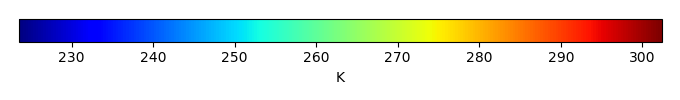MODEL MEANBIAS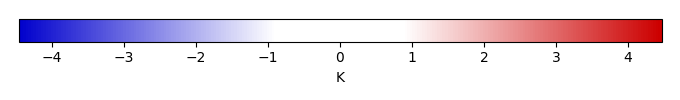BIAS SCORERMSE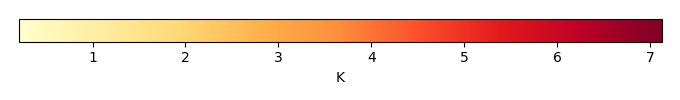RMSE SCOREBENCHMARK INTERANNUAL VARIABILITY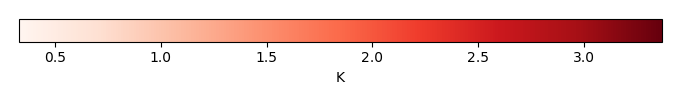MODEL INTERANNUAL VARIABILITYINTERANNUAL VARIABILITY SCOREBENCHMARK MAX MONTHMODEL MAX MONTHDIFFERENCE IN MAX MONTH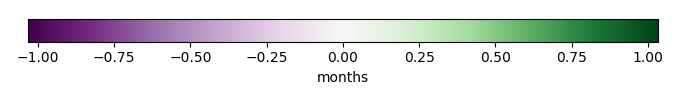SEASONAL CYCLE SCORESPATIAL TAYLOR DIAGRAMMODEL COLORS# Spatially integrated regional mean

MODEL COLORSREGIONAL MEANANNUAL CYCLEMONTHLY ANOMALYANNUAL CYCLE# All Models

BenchmarkCLM5PHSOFFCLM5PHSON# Data Information

creation_date: Fri Nov 1 11:52:30 PDT 2013

source_file: This product is generated from monthly CRU TS3.21 Mean Temperature observations

title: derived CRU high resolution (0.5x0.5) Mean Temperature

Approach: I simply read the monthly surface air temperature from original file, and separated the time series by a month and saved them by each month and each year.

Temporal resolution: monthly

General information: This product was derived from CRU v3.21 product.

Spatial resolution: 0.5x0.5 degree